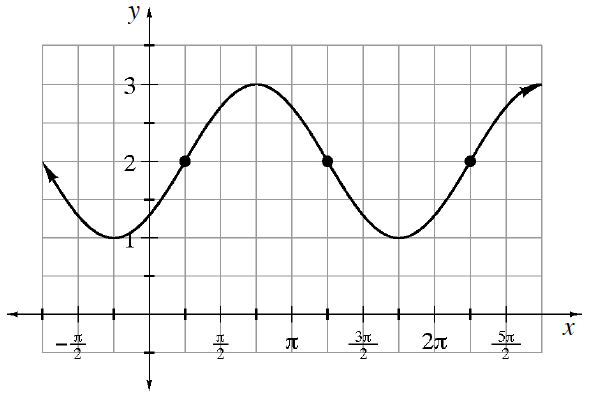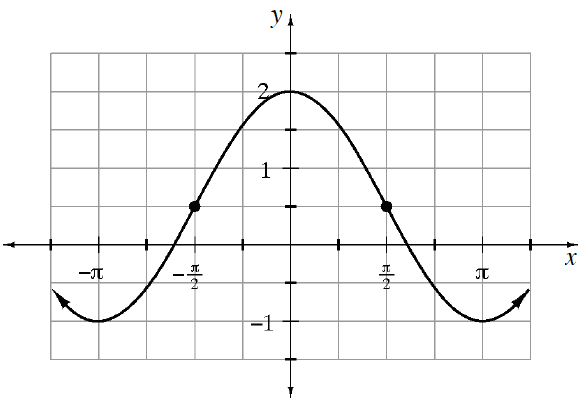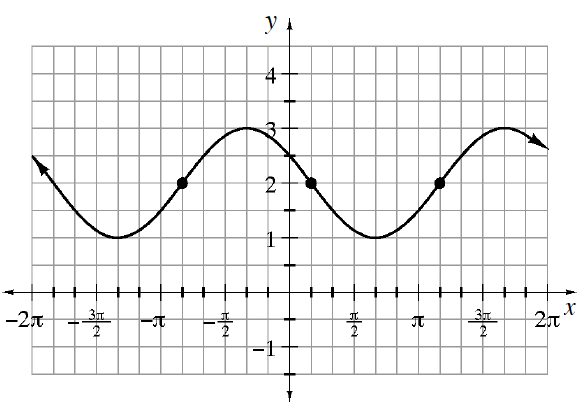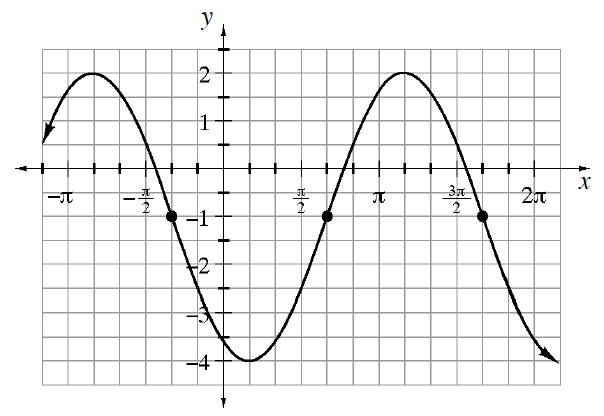### Home > INT3 > Chapter 10 > Lesson 10.1.1 > Problem10-13

10-13.

Write a possible equation for each graph.

1.Use the general equation $y = a · \text{sin} b\left(x − h\right) + k$.
Determine the value for each of the four parameters.

Identify a convenient locator point.
In this case, we will use:

$\left(\frac{\pi}{4}, 2\right)$

Since our point is on the midline, we will use the sine function.
The $x$-value of the point also represents the horizontal shift:

$h=\frac{\pi}{4}$

Next find $k$, the vertical shift.
The graph $y = \text{sin}\left(x\right)$ has a midline at $y = 0$ (the $x$-axis).
The parameter $k$ is the distance that midline is shifted vertically.
In this case $k = 2$.

The amplitude ($a$) is the distance from the midline ($k$) to the highest point.
In this case $k = 2$ and the highest point is $3$, so $a = 1$.
Since the graph increases from the locator point in the same way $y = \text{sin}\left(x\right)$ does, $a$ is positive.

You will learn more about the parameter $b$ in future lessons.
For now, know that $b = 1$ since the distance from the beginning to the end of one cycle is $2π$.

1.See part (a).
Pay careful attention to the scale on the $y$-axis when determining $a$ and $k$.

1.$\text{If you choose } \left(\frac{\pi}{6},0\right) \text{ as your locator point,}$

notice the first cycle of the graph is an inverted sine curve, so the $a$-value will be negative.

See part (a).

$y=-\sin\left(x-\frac{\pi}{6}\right)+2\text{ or }y=\sin\left(x+\frac{5\pi}{6}\right) +2$

1.See parts (a) and (c).